×

Search anything:

# Data Science Cheatsheet / List of all Data Science topics

#### data science checklistGet this book -> Problems on Array: For Interviews and Competitive Programming

This is the most complete Data Science Cheatsheet which you should follow to revise all Data Science concepts within 30 minutes and get ready for Interviews and stay in form.Data science lifecycle
PhaseDescription
Discovery
• This is where we define and understand the problem.
• Involves asking the right questions and determining all the required factors.
• Understanding dataInvolves describing what data is needed, how relevant are they and finally extracting the required data.
Data preparation
• Filter out the data applicable for the problem.
• Remove outliers
• Treat missing values
• ELiminate inaccurate data
• Merge different datasets
• Data analysis
• EDA is done here.
• We get an idea of what features to consider for building our machine learning model
• Model planningDecide on our machine learning model based on the business problem.
Model building and deploymentCreate and evaluate the ML model and finally deploy it in the preferred environment.
Communication of results
• Reflect back to our original goal that we set in the first phase
• Communicate our findings to the stakeholders
• Machine Learning
Supervised LearningType of machine learning technique where models are trained using labeled data as inputs. Commonly used fore regression and classification tasks.
Unsupervised LearningType of machine learning technique where models are trained using unalbeled data as inputs. Used for extracting information from large amounts of data.
Semi-supervised LearningCombination of supervised and unsupervised learning where a small amount of inputs are labeled and large portions of them are unlabeled.
Reinforcement LearningThis is a machine learning technique concerned with teaching agents to take decisions in environment to maximize the reward.
Regression
• These algorithms are used for finding relationships between the dependent and independent variables.
• The main goal of a regression model is to come up with an equation for the dependent variable in terms of the given independent variables.
• Classification These algorithms are used to categorize the given test data accurately, such as telling apart a cat from a dog.
Ensemble LearningEnsemble methods helps improve the performance of a machine learning model by combining several ML base models to produce one single predictive model.
Recommender Systems
• These are subset of ML that are designed to provide suggestions or recommend thing to users based on certain factors.
• It has 2 types - content based filtering, collaborative filtering.
• Supervised Learning
Logistic RegressionAn algorithm that models linear relationship between inputs and outputs a categorical variable.
• Easy to implement and interpret results.
• Efficient for unknown record classification.
• It is able to interpret coefficients as indicators of feature importance.
• Tough to obtain complex relationships.
• Assumption of linearity between input and output variables.
• May lead to overfitting when number of records are lesser than that of the features.
• Linear RegressionAn algorithm that models linear relationship between inputs and produces continuous outputs.
• Faster to train.
• Overfitting can be reduced by regularization.
• Simple to implement and performs well on linearly separable datasets.
• Assumption of linearity between input and output variables.
• Sensitive to outliers.
• Prone to underfitting.
• Support Vector MachinesAn algorithm that aims to create the best decision boundary to group n-dimensional space into different classes.
• More effective in higher dimensional spaces.
• Is effecient when number of specimens are lesser than the dimensions.
• Does not perform well with large datasets.
• Poor performance in case of noisy data
• Random ForestIt is a combination of many decision trees and is an ensemble learning method.
• Higher accuracy compared to other models
• Reduces overfitting.
• Training complexity becomes high when number of decision trees increases.
• Poor performance on imbalanced data.
• Decision TreeAn algorithm that can be used for both regression and classification where models make decision rules on features to obtain predictions.
• Can handle missing values
• Can handle multi-output problems.
• Of ten relatively inaccurate compared to other predictors.
• Small change in data can cause a huge change in its structure.
• K-Nearest NeighborsAn algorithm that uses feature similarity to predict values of new data points.
• Evolves with new data point.
• Is capable of learning non-linear functions.
• No explicit training time.
• Complexity of prediction increases with increase in number of dimensions.
• Assumes all features are equally important.
• Unsupervised Learning
K-Means ClusteringA clustering algorithm that determines K clusters based on euclidean distances.
• Simple to implement and understand.
• Can be scaled to large datasets.
• Outputs tight clusters.
• Number of clusters are to be specified in the beginning.
• Has problem when data has clusters of varying densities and sizes.
• Hierarchical Clustering
• Each data item is treated as a single cluster and two closest clusters are successively merged together.
• Bottom-up approach.
• Results in a highly informative dendrogram.
• Need not specify number of clusters at the start.
• Not suitable for highly complex and large datasets.
• Does not always result in best clusters.
• DBSCAN
• It is a density based clustering algorithm.
• Clusters are highly dense regions in space separated by regions of lower densities.
• Need not specify number of clusters at the start.
• Supports non-globular cluster shapes.
• Does not perform well for high dimensional data.
• Fails when differences between the densities of clusters are too large.
• Apriori Algorithm
• Most frequent set of items in a dataset are indentified with prior knowledge of theor properties.
• Is a rule based approach
• Produces intuitive and easy-to-understand results.
• Can be easily parallelized.
• Generates many unwanted itemsets
• Computationally complex
• Memory intensive
• Principal Component AnalysisThis algorithm is widely used for dimensionality reduction.
• Easy to compute.
• Prevents the issues of using high dimensional data
• Trade-off between reducing dimensions and information loss.
• Principal components are not easy to interpret.
• Manifold LearningIt is used for non-linear dimensionality reduction and aims to describe datasets as low-dimensional manifolds embedded in high-dimensional spaces.
• Preserve non-linear relationships in data.
• No good framework for handling missing data.
• Noise in data can affect the embedding highly
• Deep Learning
Neural NetworkA neural network takes an input, passes it through multiple layers of hidden neurons and outputs a prediction representing the combined input of all the neurons.
Architectures
• CNN - CNN has neurons that can receive many inputs, takes the weighted sum of each neuron's input and passes it through an activation function. There is also a loss function associated to it at the end
• RNN - In RNN, output from previous step are fed as input to the current step.
• GAN - A GAN has 2 main components: a generator model and a discriminator model. These models learn the patterns in the input data in such a way that it is able to generate output samples that likely belong to the original dataset.
• MLP - A MLP is a neural network with only fully connected layers.
• Autoencoder - Auto-encoders are learning networks that gets the input, encodes them and then learn to reconstruct the data from the encoded form to an output that is as close to the input as possible.
• LSTMLSTM is a variant of RNN that is used for learning long term dependencies. It has a memory cell to record additional information.
Back propagationA back propagation algorithm consists of two main steps:
• Feed forward the values
• Calculate the error and propagate it back to the layers before.
• Gradient descent is an optimization algorithm used to find values of parameters of an activation function that minimizes the function.
• Basically, it measures the amount of change in the output function when the inputs are changed a little bit.
• Activation function
• Activation functions decide whether the neuron should be activated or not i.e. if the neuron's input is important or not.
• ReLU and sigmoid are commonly used activation functions.
• Loss function
• Measures how well the network models the given training data.
• Compares the predicted and target output values.
• Optimizers
• They update the model in response to the output of the loss function by tweaking the weights.
• RegularizationIt is a technique for combating overfitting and improving training. Some of them are early stopping, data augmentation and ensembling.
Layers
• Convolution - This layer performs the convolution operation i.e different feature maps are convoluted over the dataset.
• Pooling - This layer reduces the dimensionality of the stack of outputs from the activation layer.
• Batch Normalization - The batch norm layer normalizes the incoming activations and outputs a new batch where the mean equals 0 and standard deviation equals 1. It subtracts the mean and divides by the standard deviation of the batch.
• Fully connected layer - This layer predicts the image and classifies objects in it.
• Dropout - A dropout layer takes the output of the previous layer’s activations and randomly sets a certain fraction (dropout rate) of the activated neurons to 0, cancelling or ‘dropping’ them out.
• Python Basics
ConceptCodeDescription
NumPy
Creating arrays
• a = np.array([1,2,3])
• b = np.array([(1.5,2,3), (4,5,6)], dtype = float)
• Inspecting the array
• a.shape
• len(a)
• b.ndim
• b.size
• b.dtype
• Array dimensions
• Length of array
• Number of array dimensions
• Number of array elements
• Data type of array elements
• Arithmetic Operations
• np.subtract(a,b)
• np.divide(a,b)
• np.multiply(a,b)
• np.sqrt(b)
• Subtraction
• Division
• Multiplication
• Square root
• Aggregate functions
• a.sum()
• a.min()/a.max()
• b.cumsum(axis=1)
• a.mean()
• a.corrcoef()
• np.std(b)
• b.median()
• Array-wise sum
• Array-wise minimum/maximum value
• Cumulative sum of the elements
• Mean
• Correlation coefficient
• Standard deviation
• Median
• Subsetting and Slicing
• a
• b[1,2]
• a[0:2]
• b[0:2,1]
• b[:1]
• a[ : :-1]
• Select the element at the 2nd index
• Select the element at row 0 column 2
• Select items at index 0 and 1
• Select items at rows 0 and 1 in column 1
• Select all items at row 0 (same as b[0:1, :])
• Reversed array a
• Array manipulation
• np.transpose(b)
• b.ravel()
• b.resize((2,4))
• np.append(a,b)
• np.insert(a, 1, 5)
• np.delete(a,)
• a.sort()
• Transpose array
• Flatten the array
• Return a new array with shape (2,4)
• Append items to an array
• Insert items in an array
• Delete items from an array
• Sort an array
• Pandas
Seriess = pd.Series([3, -5, 7, 4], index=['a', 'b', 'c', 'd'])A one-dimensional labeled array a capable of holding any data type
DataFramedata = {'Country': ['Belgium', 'India', 'Brazil'], 'Capital': ['Brussels', 'New Delhi', 'Brasília'], 'Population': [11190846, 1303171035, 207847528]}
df = pd.DataFrame(data, columns=['Country', 'Capital', 'Population'])
A two-dimensional labeled data structure with columns of potentially different types
Selecting and setting
• df.iloc(,) / df.iat(,)
• df.loc(, ['Country']) / df.at(, ['Country'])
• df.ix[:,'Capital']
• df[df['Population']>1200000000]
• s['a'] = 6
• Select single value by row & column
• Select single value by row & column labels
• Select a single column of a set of columns
• Use filter to adjust DataFrame
• Set index a of Series s to 6
• Sorting and dropping
• df.sort_index()
• df.sort_values(by='Country')
• s.drop(['a', 'c'])
• df.drop('Country', axis=1)
• Sort by labels along an axis
• Sort by the values along an axis
• Drop values from rows (axis=0)
• Drop values from columns(axis=1)
• Retrieving basic dataframe information
• df.shape
• df.index
• df.columns
• df.info()
• df.count
• (rows,columns)
• Describe index
• Describe DataFrame columns
• Info on DataFrame
• Number of non-NA values
• Summary of dataframe information
• df.sum()
• df.cumsum()
• df.min()/df.max()
• df.describe()
• df.mean() / df.median()
• Sum of values
• Cummulative sum of values
• Minimum/maximum values
• Summary statistics
• Mean/median of values
• Statistics and Probability
ConceptDescriptionFormula/Graph
MeanThe mean denotes the average of the group of finite numbers.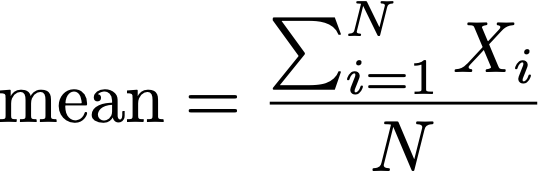MedianThe median denotes the middle of an ordered set of data.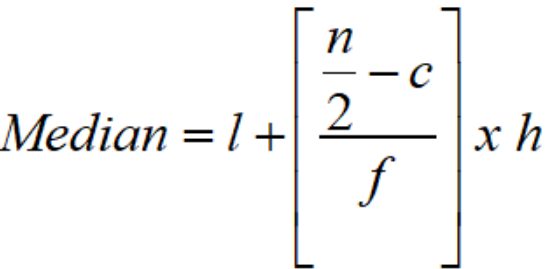ModeIs only relevant for discrete data and is the the most common value occurring in a dataset.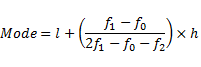VarianceVariance gives a measure of the degree to which each value in the population/sample differs from the mean value.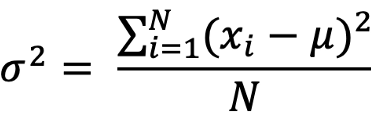Standard deviationThe standard deviation tells us how much the values in the sample/ population is spread out from the mean value.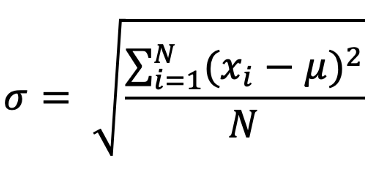CovarianceCovariance is used to identify how they both change together and also the relationship between them.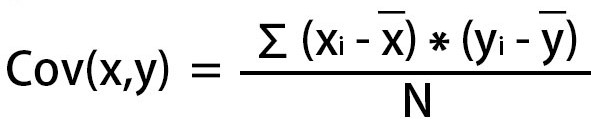CorrelationCorrelation is dimensionless and is used to quantify the relationship between two variables. It has its range as [-1,1].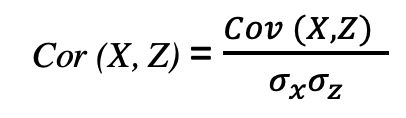Central limit theoremIt states that "As the sample size becomes larger, the distribution of sample means approximates to a normal distribution curve."
Law of large numbersThe law of large numbers states that As the number of trials or observations increases, the actual or observed average approaches the theoretical or expected average.
Bayes theorem
• The probability of X given Y is equal to the probability of Y given X multiplied by the probability of X over the probability of Y
• Based on conditional probability
•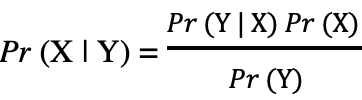Hypothesis testing
• It helps us identify whether an action should be performed or not based on the results it will yield.
• In hypothesis testing, we usually consider two hypotheses: Null and alternative.
• A/B testingA/B testing is a famous testing technique used to compare two variants to determine the best of the two based on user experience.
Confidence intervalsA Confidence interval expresses a range of values within which we are pretty sure that the population parameter lies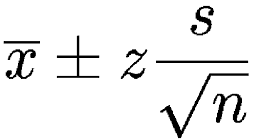Normal distribution
• Known as the bell-curve.
• Has mean=0 and standard deviation=1.
•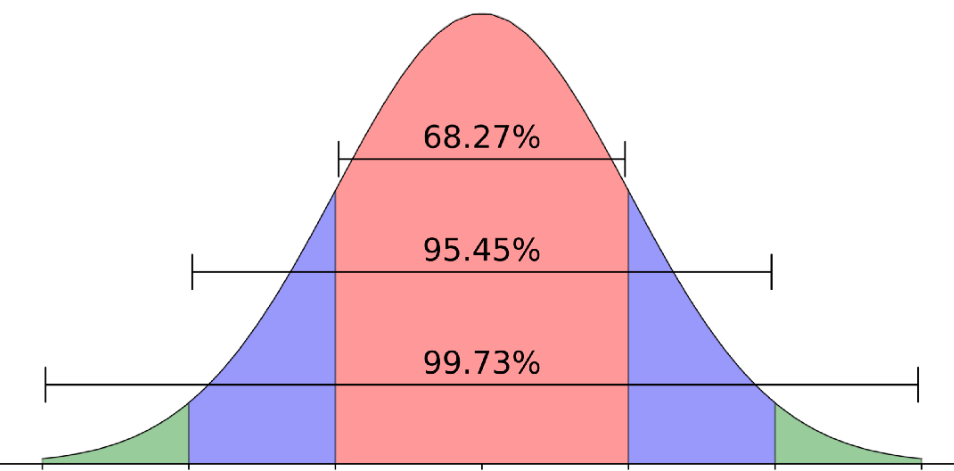Poisson distributionDistribution that expresses the probability of a given number of events occurring within a fixed time period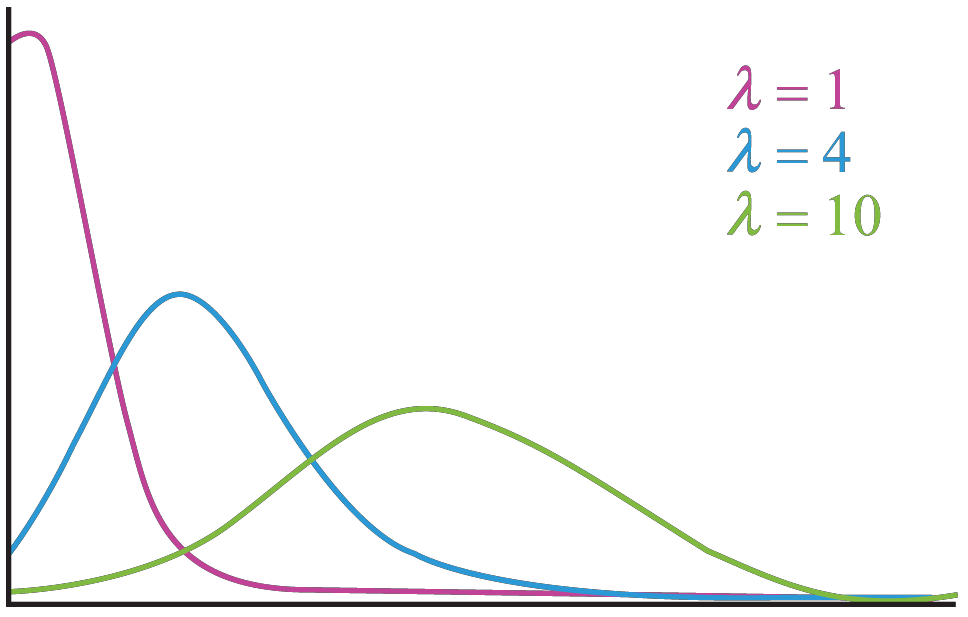Data visualization
ChartDescriptionImage
Capturing trends
Line chart
• Capture how a numeric variable is changing over time.
• May contain one or many lines depending on variables.
•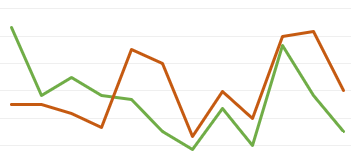Area chart
• Shows progression of a numeric value with shaded area between line and the x-axis.
• May be stacked.
•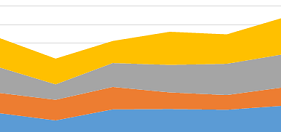Capturing distributions
Histogram
• Shows the distribution of a variable.
• The x-axis shows the range, and the y-axis represents the frequency.
•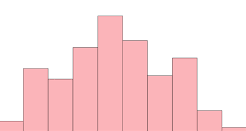BoxplotShows the distribution of a variable using 5 key summary statistics.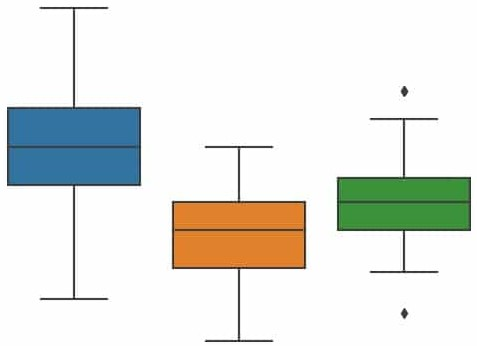Violinplot
• A variation of the box plot.
• It also shows the full distribution of the data alongside summary statistics
•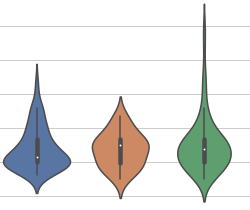Part to-whole charts
Pie chart
• Most common way to visualize part to whole data.
• It is also commonly used with percentages.
•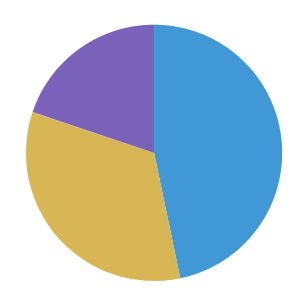Donut chart
• Variant of pie chart
• It has a hole in middle for readability.
•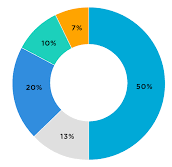Heatmap
• 2 dimensional chart
• Use colors to represent data trends.
•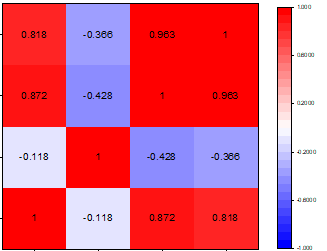Stacked chartCompare subcategories within categorical data.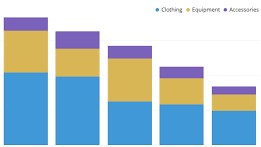Visualising relationships
Bar/column chart
• Ouick comparison of categorical variables
• One axis contains categories and the other axis represents values
•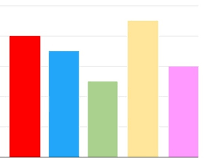Scatter plot
• Observing relationship between 2 variables.
• Useful for quickly surfacing potential correlations between data points
•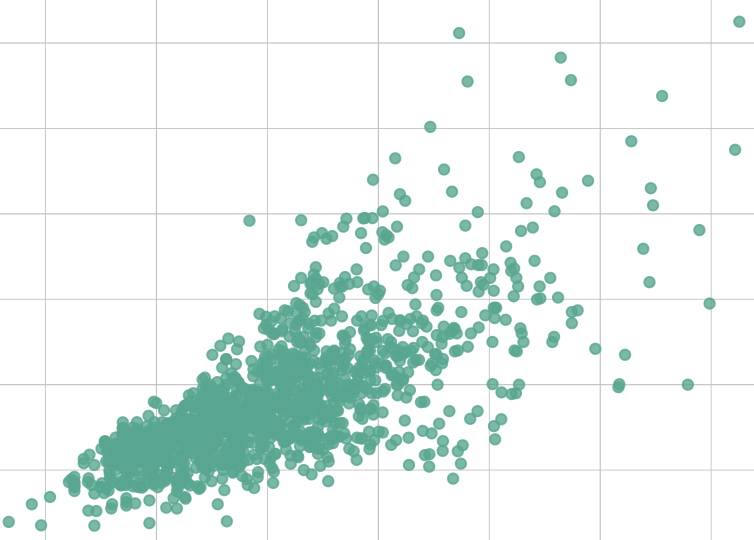Bubble chart
• Visualize data points with 3 dimensions.
• It tries to show relations between data points using location and size
•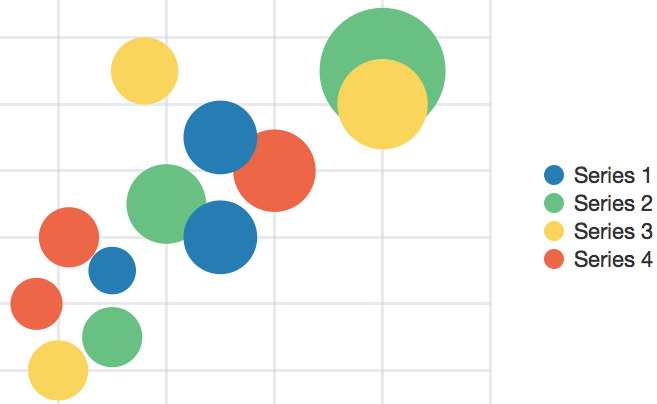Time series analysis
ConceptDescriptionCode
ACF plotThe autocorrelation function (ACF) plot shows the autocorrelation coefficients as a function of the lag.
• We can use it to determine the order q of a stationary MA(q) process
• import statsmodels.api as sm
sm.graphics.tsa.plot_acf(data)
PACF plotThe partial autocorrelation function (PACF) plot shows the partial autocorrelation coefficients as a function of the lag.
• We can use it to determine the order p of a stationary AR(p) process
• import statsmodels.api as sm
sm.graphics.tsa.plot_pacf(data)
ADF testIf a series is stationary, its mean, variance, and autocorrelation are constant over time. We can test for stationarity with augmented Dickey-Fuller (ADF) test.
• Null hypothesis: the series is not stationary
• We want a p-value less than 0.05
Time series decompositionSeparate the series into 3 components: trend,seasonality, and residuals
• Trend: long-term changes in the series
• Seasonality: periodical variations in the series
• Residuals: what is not explained by trend and seasonality
• from statsmodels.tsa.seasonal import STL
decomp=STL(data,period=m).fit()
plt.plot(decomp.observed)
plt.plot(decomp.trend)
plt.plot(decomp.seasonal)
plt.plot(decomp.resid)
Moving average model – MA(q)The moving average model: the current value depends on the mean of the series, the current error term, and past error terms.
• Denoted as MA(q) where q is the order
• Use ACF plot to find q
• Assumes stationarity. Use only on stationary data
• from statsmodels.tsa.statespace.sarimax import SARIMAX
model=SARIMAX(data,order=(0,0,q))
Autoregressive model – AR(p)The autoregressive model is a regression against itself. This means that the present value depends on past values.
• Denoted as AR(p) where p is the order
• Use PACF to find p
• Assumes stationarity. Use only on stationary data
• from statsmodels.tsa.statespace.sarimax import SARIMAX
model=SARIMAX(data,order=(p,0,0))
ARMA(p,q)The autoregressive moving average model (ARMA) is the combination of the autoregressive model AR(p), and the moving average model MA(q).
• Denoted as ARMA(p,q) where p is the order of the autoregressive portion, and q is the order of the moving average portion
• Cannot use ACF or PACF to find the order p, and q. Must try different (p,q) value and select the model with the lowest AIC (Akaike’s Information Criterion)
• Assumes stationarity. Use only on stationary data
• from statsmodels.tsa.statespace.sarimax import SARIMAX
model=SARIMAX(data,order=(p,0,q))
ARIMA(p,d,q)The autoregressive integrated moving average (ARIMA) model is the combination of the autoregressive model AR(p), and the moving average model MA(q), but in terms of the differenced series.
• Denoted as ARMA(p,d,q), where p is the order of the autoregressive portion, d is the order of integration, and q is the order of the moving average portion
• Can use on non-stationary data
• from statsmodels.tsa.statespace.sarimax import SARIMAX
model=SARIMAX(data,order=(p,d,q))
SARIMA(p,d,q)(P,D,Q)mThe seasonal autoregressive integrated moving average (SARIMA) model includes a seasonal component on top of the ARIMA model.
• Denoted as SARIMA(p,d,q)(P,D,Q)m. Here, p, d, and q have the same meaning as in the ARIMA model.
• P,D, and Q are the seasonal orders of autoregressive, integrated and moving average portions.
• m is the frequency of the data (i.e., the number of data points in one season)
• from statsmodels.tsa.statespace.sarimax import SARIMAX
model=SARIMAX(data,order=(p,d,q),seasonal_order=(P,D,Q,m))#### Sanjana Babu

Sanjana Babu is a Student at SRM Valliammai Engineering College and is an Intern at OpenGenus. She is a Philomath.